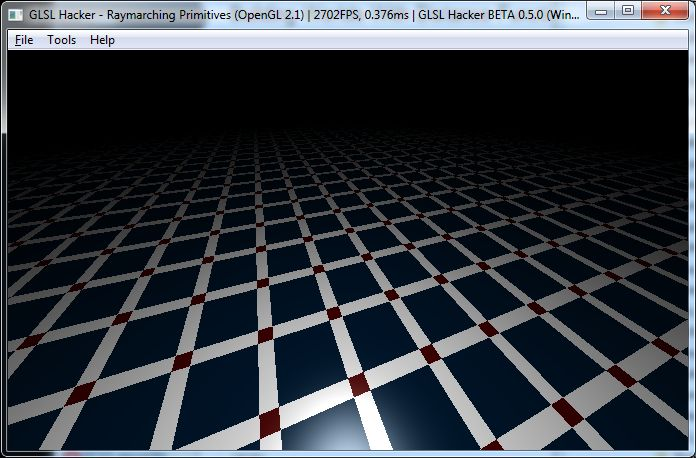# Building Worlds With Distance Functions in GLSL (Raymarching)

Article index:

## 2 – GLSL framework for Raymarching

So what do we need to create worlds with raymarching? It’s really simple: a quad (two triangles) and a GLSL program made up of one vertex shader and one pixel shader. This GLSL program is used during the rendering of the quad. That’s all!Raymarching: the floor!

Here is the base code to get the image right above: a raymarched floor. All following raymarched shaders will update the distance_to_obj() function. That’s all…

```void main()
{
gl_TexCoord = gl_MultiTexCoord0;
gl_Position = ftransform();
}
```

```uniform vec3 cam_pos;
uniform float time;
uniform vec2 resolution;

//uniform vec2 mouse;
float PI=3.14159265;

// Floor
vec2 obj_floor(in vec3 p)
{
return vec2(p.y+10.0,0);
}

//Objects union
vec2 distance_to_obj(in vec3 p)
{
return obj_floor(p);
}

//Floor Color (checkerboard)
vec3 floor_color(in vec3 p)
{
if (fract(p.x*0.2)>0.2)
{
if (fract(p.z*0.2)>0.2)
return vec3(0,0.1,0.2);
else
return vec3(1,1,1);
}
else
{
if (fract(p.z*.2)>.2)
return vec3(1,1,1);
else
return vec3(0.3,0,0);
}
}

// Primitive color
vec3 prim_c(in vec3 p)
{
return vec3(0.6,0.6,0.8);
}

void main(void)
{
vec2 q = gl_TexCoord.xy;
vec2 vPos = -1.0 + 2.0 * q;

// Camera up vector.
vec3 vuv=vec3(0,1,0);

// Camera lookat.
vec3 vrp=vec3(0,0,0);

//float mx=mouse.x*PI*2.0;
//float my=mouse.y*PI/2.01;
//vec3 prp=vec3(cos(my)*cos(mx),sin(my),cos(my)*sin(mx))*6.0;
vec3 prp = cam_pos;

// Camera setup.
vec3 vpn=normalize(vrp-prp);
vec3 u=normalize(cross(vuv,vpn));
vec3 v=cross(vpn,u);
vec3 vcv=(prp+vpn);
//vec3 scrCoord=vcv+vPos.x*u*resolution.x/resolution.y+vPos.y*v;
vec3 scrCoord=vcv+vPos.x*u*0.8+vPos.y*v*0.8;
vec3 scp=normalize(scrCoord-prp);

// Raymarching.
const vec3 e=vec3(0.02,0,0);
const float maxd=100.0; //Max depth
vec2 d=vec2(0.02,0.0);
vec3 c,p,N;

float f=1.0;
for(int i=0;i<256;i++)
{
if ((abs(d.x) < .001) || (f > maxd))
break;

f+=d.x;
p=prp+scp*f;
d = distance_to_obj(p);
}

if (f < maxd)
{
// y is used to manage materials.
if (d.y==0)
c=floor_color(p);
else
c=prim_c(p);

vec3 n = vec3(d.x-distance_to_obj(p-e.xyy).x,
d.x-distance_to_obj(p-e.yxy).x,
d.x-distance_to_obj(p-e.yyx).x));
N = normalize(n)
float b=dot(N,normalize(prp-p));
//simple phong lighting, LightPosition = CameraPosition
gl_FragColor=vec4((b*c+pow(b,16.0))*(1.0-f*.01),1.0);
}
else
gl_FragColor=vec4(0,0,0,1); //background color
}
```

This GLSL framework takes its source from this demo and this demo by Paulo Falcao.

Article index:

## 4 thoughts on “Building Worlds With Distance Functions in GLSL (Raymarching)”

1.GLSLnoob42

Hey. Just found this. Came across iq’s stuff a while ago, but being a noob I couldn’t do anything with it.

Then while rediscovering GLSL & iq’s website, I found this awesome resource!

But here’s the thing, I can’t get it to work. ;o)

I eventually found 2 errors in the code. Here are the fixes:
line 97: d.x-distance_to_obj(p-e.yyx).x); // had an extra “)”
line 98: N = normalize(n); // was missing “;”

I’m trying to use GLSL Hacker 0.6.0.0 & Blender 2.69 using this tutorial:

Any help?

peace & 42

2.toba

I too found the errors but cant get any of this to work. There are too few good examples online, it would be nice to get one working

3.JeGX Post Author

I’m fixing the errors and I upload the code samples asap!